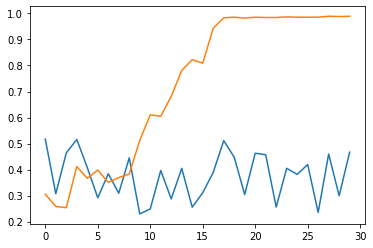# Search Algorithms¶

## AutoGluon System Implementation Logic¶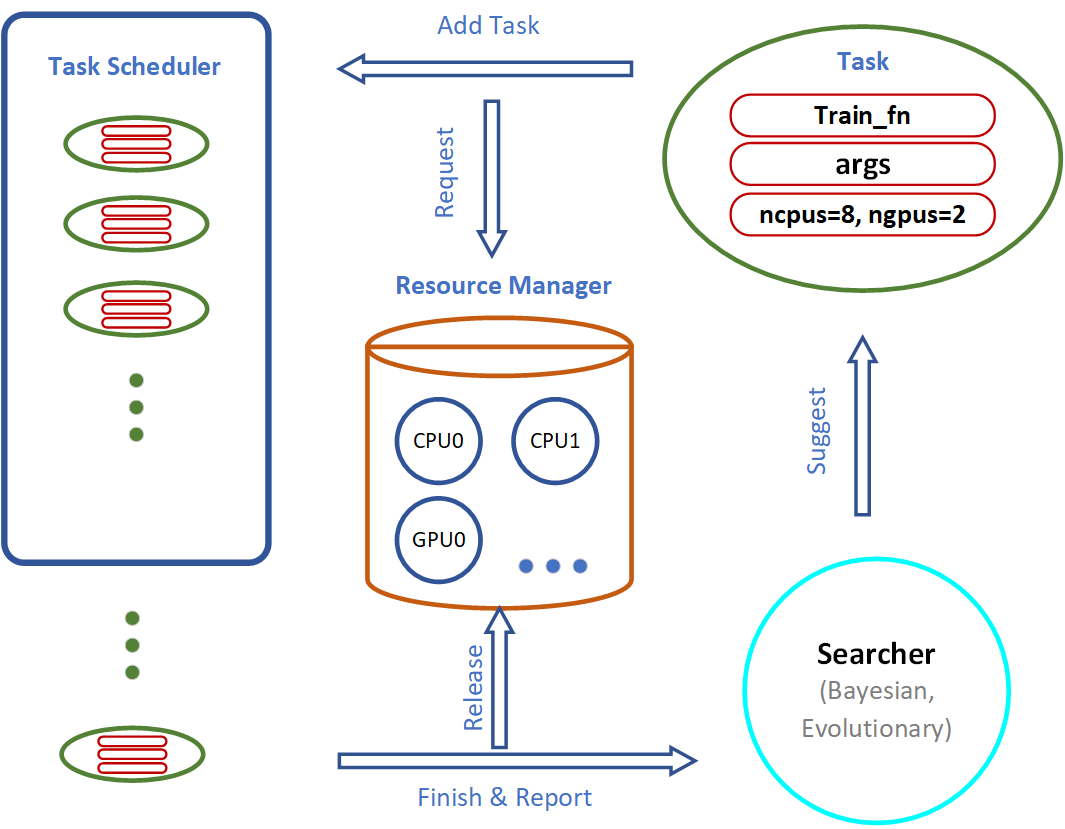Important components of the AutoGluon system include the Searcher, Scheduler and Resource Manager:

• The Searcher suggests hyperparameter configurations for the next training job.

• The Scheduler runs the training job when computation resources become available.

In this tutorial, we illustrate how various search algorithms work and compare their performance via toy experiments.

## FIFO Scheduling vs. Early Stopping¶

In this section, we compare the different behaviors of a sequential First In, First Out (FIFO) scheduler using `autogluon.core.scheduler.FIFOScheduler` vs. a preemptive scheduling algorithm `autogluon.core.scheduler.HyperbandScheduler` that early-terminates certain training jobs that do not appear promising during their early stages.

### Create a Dummy Training Function¶

```import numpy as np
import autogluon.core as ag

@ag.args(
lr=ag.space.Real(1e-3, 1e-2, log=True),
wd=ag.space.Real(1e-3, 1e-2),
epochs=10)
def train_fn(args, reporter):
for e in range(args.epochs):
dummy_accuracy = 1 - np.power(1.8, -np.random.uniform(e, 2*e))
reporter(epoch=e+1, accuracy=dummy_accuracy, lr=args.lr, wd=args.wd)
```

### FIFO Scheduler¶

This scheduler runs training trials in order. When there are more resources available than required for a single training job, multiple training jobs may be run in parallel.

```scheduler = ag.scheduler.FIFOScheduler(train_fn,
resource={'num_cpus': 2, 'num_gpus': 0},
num_trials=20,
reward_attr='accuracy',
time_attr='epoch')
scheduler.run()
scheduler.join_jobs()
```
```HBox(children=(HTML(value=''), FloatProgress(value=0.0, max=20.0), HTML(value='')))
```

Visualize the results:

```scheduler.get_training_curves(plot=True, use_legend=False)
```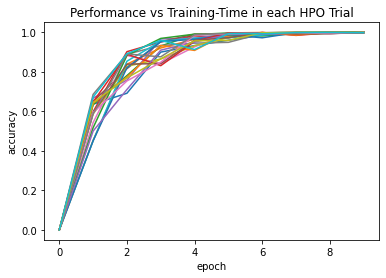### Hyperband Scheduler¶

AutoGluon implements different variants of Hyperband scheduling, as selected by `type`. In the `stopping` variant (the default), the scheduler terminates training trials that don’t appear promising during the early stages to free up compute resources for more promising hyperparameter configurations.

```scheduler = ag.scheduler.HyperbandScheduler(train_fn,
resource={'num_cpus': 2, 'num_gpus': 0},
num_trials=100,
reward_attr='accuracy',
time_attr='epoch',
grace_period=1,
reduction_factor=3,
type='stopping')
scheduler.run()
scheduler.join_jobs()
```
```HBox(children=(HTML(value=''), FloatProgress(value=0.0), HTML(value='')))
```

In this example, trials are stopped early after 1, 3, or 9 epochs. Only a small fraction of the most promising jobs run for the full number of 10 epochs. Since the majority of trials are stopped early, we can afford a larger `num_trials`. Visualize the results:

```scheduler.get_training_curves(plot=True, use_legend=False)
```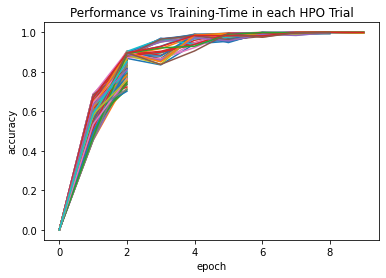Note that `HyperbandScheduler` needs to know the maximum number of epochs. This can be passed as `max_t` argument. If it is missing (as above), it is inferred from `train_fn.args.epochs` (which is set by `epochs=10` in the example above) or from `train_fn.args.max_t` otherwise.

## Random Search vs. Reinforcement Learning¶

In this section, we demonstrate the behaviors of random search and reinforcement learning in a simple simulation environment.

### Create a Reward Function for Toy Experiments¶

Import the packages:

```import matplotlib.pyplot as plt
from mpl_toolkits.mplot3d import Axes3D
```

Input Space `x = [0: 99], y = [0: 99]`. The rewards is a combination of 2 gaussians as shown in the following figure:

Generate the simulated reward as a mixture of 2 gaussians:

```def gaussian2d(x, y, x0, y0, xalpha, yalpha, A):
return A * np.exp( -((x-x0)/xalpha)**2 -((y-y0)/yalpha)**2)

x, y = np.linspace(0, 99, 100), np.linspace(0, 99, 100)
X, Y = np.meshgrid(x, y)

Z = np.zeros(X.shape)
ps = [(20, 70, 35, 40, 1),
(80, 40, 20, 20, 0.7)]
for p in ps:
Z += gaussian2d(X, Y, *p)
```

Visualize the reward space:

```fig = plt.figure()
ax = fig.gca(projection='3d')
ax.plot_surface(X, Y, Z, cmap='plasma')
ax.set_zlim(0,np.max(Z)+2)
plt.show()
```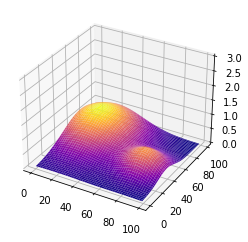### Create Training Function¶

We can simply define an AutoGluon searchable function with a decorator `ag.args`. The `reporter` is used to communicate with AutoGluon search and scheduling algorithms.

```@ag.args(
x=ag.space.Categorical(*list(range(100))),
y=ag.space.Categorical(*list(range(100))),
)
def rl_simulation(args, reporter):
x, y = args.x, args.y
reporter(accuracy=Z[y][x])
```

### Reinforcement Learning¶

```rl_scheduler = ag.scheduler.RLScheduler(rl_simulation,
resource={'num_cpus': 1, 'num_gpus': 0},
num_trials=300,
reward_attr="accuracy",
controller_batch_size=4,
controller_lr=5e-3)
rl_scheduler.run()
rl_scheduler.join_jobs()
print('Best config: {}, best reward: {}'.format(rl_scheduler.get_best_config(), rl_scheduler.get_best_reward()))
```
```100%|██████████| 76/76 [00:19<00:00,  3.89it/s]
```
```Best config: {'x▁choice': 21, 'y▁choice': 74}, best reward: 0.9892484241569526
```

### Compare the performance¶

Get the result history:

```results_rl = [v['accuracy'] for v in rl_scheduler.training_history.values()]
results_random = [v['accuracy'] for v in random_scheduler.training_history.values()]
```

Average result every 10 trials:

```import statistics
results1 = [statistics.mean(results_random[i:i+10]) for i in range(0, len(results_random), 10)]
results2 = [statistics.mean(results_rl[i:i+10]) for i in range(0, len(results_rl), 10)]
```

Plot the results:

```plt.plot(range(len(results1)), results1, range(len(results2)), results2)
```
```[<matplotlib.lines.Line2D at 0x7ffaa5811d10>,
<matplotlib.lines.Line2D at 0x7ffb68884a90>]
```